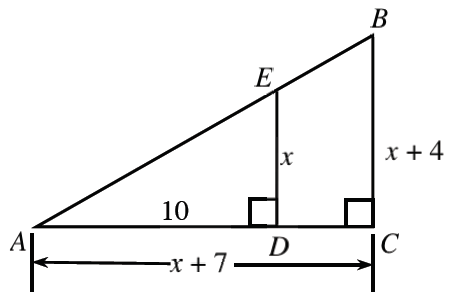### Home > A2C > Chapter 2 > Lesson 2.1.4 > Problem2-48

2-48.

In the diagram at right, $ΔABC∼ΔAED$.

1. Solve for $x$.

The sides of the triangle are proportional. Write an equation and solve for $x$.

Because distance cannot be negative, $x=8$, not $x=−5$.

2. Calculate the perimeter of $ΔAED$.

Use your results from part (a) and the Pythagorean Theorem to determine the length of the hypotenuse.